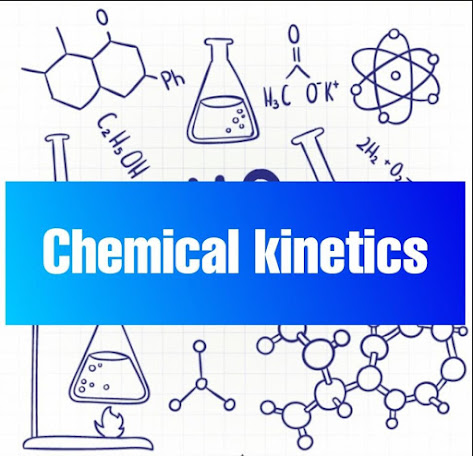chemical kineticsIntroduction

substances with well-defined properties are converted by chemical reactions into other substances with different properties for any chemical reaction chemistry tries to find out the feasibility of a chemical reaction which can be predicted by thermodynamics and the extent to which a reaction will proceed can be determined from chemical equilibrium speed of a reaction that is time taken by a reaction to reach equilibrium rate of a chemical reaction the rate of a reaction can be defined as the change in concentration of a reactant in

or product in unit time consider a hypothetical reaction assuming that the volume of the system remains constant R is 2 P 1 mole of the reactant R produces 1 mole of the product P if R 1 and P 1 are the concentrations of R and P respectively at time T 1 and R 2 and P 2 are their concentrations at time T 2 then it is calculated as above equation 1 and 2 given above represent the average rate of reaction the instantaneous and average rate of reaction are given

A and B respectively factors influencing rate reaction rate of a reaction depends upon the experimental conditions such as concentration of reactants temperature and catalyst the factors shown above such as dependence on concentration rate expression and

rate constant order and molecularity also influence the rate of a reaction order of a reaction the sum of powers of the concentration of the reactants in the rate law expression is called the order of death chemical reaction the order of a reaction can be 0 1 2 3 and even a fraction as zero order reaction means that the rate of reaction is independent of the concentration of reactants the units of rate constants first-order reaction a first-order reaction depends on the concentration of only one reactant other reactants can be present

but each will be zero order the rate law for an elementary reaction that is first order with respect to a reactant a is shown above where K is the first order rate constant which has units of one time examples of reactions that are first order with respect to the reactant second-order reaction a second-order reaction depends on the concentrations of one second-order reactant or two

first-order reactants for a second-order reaction its reaction rate half-life of a reaction half-life period t1 by 2 of a reaction is defined as the time required to reduce the concentration of a reactant to 1/2 of its initial value T 1 by 2 values are calculated by using the integrated rate equation of any order of a reaction

thus half-life period of a first-order reaction is independent of the initial concentration of the reactant and also inversely proportional to the rate constant of a reaction temperature dependence of the rate of a reaction most of the chemical reactions are accelerated by increase in temperature it has been found that for a chemical reaction with rise in temperature by 10 the rate constant is nearly doubled the temperature dependence of the rate of a chemical reaction can be accurately explained by

our genius equation according to our genius this reaction can take place only when a molecule of hydrogen and a molecule of iodine collide to form an unstable intermediate figure it exists for a very short time and then breaks up to form 2 molecules of hydrogen iodide

the figure is obtained by plotting potential energy versus reaction coordinate reaction coordinate represents the profile of energy change when reactants change into products some energy is released when the complex decomposes to form products so the final heat of the reaction depends upon the nature of reactants and products the distribution of kinetic energy may be described by plotting the fraction of molecules with a given kinetic energy E

versus kinetic energy figure here n E is the number of molecules with energy E and n T is total number of molecules the distribution of energy may be described by plotting the fraction of molecules with a given kinetic energy versus kinetic energy figure here n E is the number of molecules with energy e and NT is total number of molecules when the temperature is raised the maximum of the curve moves to the higher energy value

and the curve brought us out that is spreads to the right such that there is a greater proportion of molecules with much higher energies the area under the curve must be constant since total probability must be 1 at all times we can mark the position of EE on maxwell-boltzmann distribution curve effect of catalyst reactions that take place slowly at room

temperature are hastened by adding another substance generally in small amounts to the reaction mixture this substance is called catalyst the catalyst is involved in the reaction but is not consumed in the reaction it increases the rate of the reaction by changing the path of the reaction the reaction takes place through a different path of lower activation energy instead of the original path of higher

activation energy in presence of the catalyst this effect is indicated graphically in the figure collision theory of reaction rates one of the most stable theories in the physical sciences is the collision theory of reaction rates according to this theory product formation can only take place when there are effective collisions between reactant molecules involved in the rate

determining step of the process simply having reactant molecules colliding is necessary but not sufficient in itself the collisions have to be effective the main postulates of the theory are shown above.

if you have any doubt regarding this topic you can comment down or dont feel hesitate to contact us at infozones.in@gmail.com.